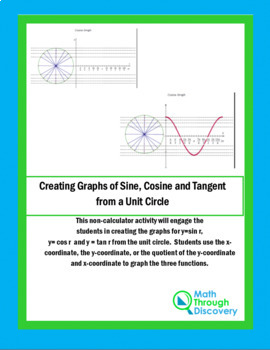Creating Graphs of Sine, Cosine, and Tangent from a Unit CircleSubject
Resource Type
Product Rating
4.0
7 Ratings
File Type

PDF (Acrobat) Document File

Be sure that you have an application to open this file type before downloading and/or purchasing.

372 KB|9 pages
Share
Also included in:
1. This bundle included four products from my store:- Graphs of Sine, Cosine, and Tangent from a Unit Circle- Building a Unit Circle- Unit Circle and Graph of Six Trigonometric Functions- Folding a Unit Circle
\$13.50
\$12.15
Save \$1.35
Product Description

This non-calculator activity will engage the students in creating the graphs for y = sin r,

y = cos r and y = tan r from the unit circle for values of 0 ≤ r ≤ 2π.

• To graph the function y = sin r students are given a unit circle (divided into sixteen parts to match with π/6, π/3, π/4, π/2, etc) adjacent to an rx plane. Using the value of r and the x coordinate for each point on the unit circle, students create a graph of thirteen points on y = sin r. They are then asked to draw a smooth curve through the thirteen points.

• To graph the function y = cos r students are given a unit circle (divided into sixteen parts to match with π/6, π/3, π/4, π/2, etc) adjacent to an rx plane. Using the value of r and the y coordinate for each point on the unit circle, students create a graph of thirteen points on y = cos r. They are then asked to draw a smooth curve through the thirteen points.

• To graph the function y = tan r students are given a unit circle (divided into sixteen parts to match with π/6, π/3, π/4, π/2, etc) adjacent to an rx plane. Using the value of r and the quotient of the y coordinate and the x coordinate for each point on the unit circle, students create a graph of thirteen points on y = tan r. They are then asked to draw a smooth curve through the thirteen points. Students observe that this graph is not a continuous graph. There is asymptotic behavior at π/2 and 3π/2.

Great discovery activity!

This is a great resource!

Total Pages
9 pages
Included
Teaching Duration
N/A
Report this Resource to TpT
Reported resources will be reviewed by our team. Report this resource to let us know if this resource violates TpT’s content guidelines.